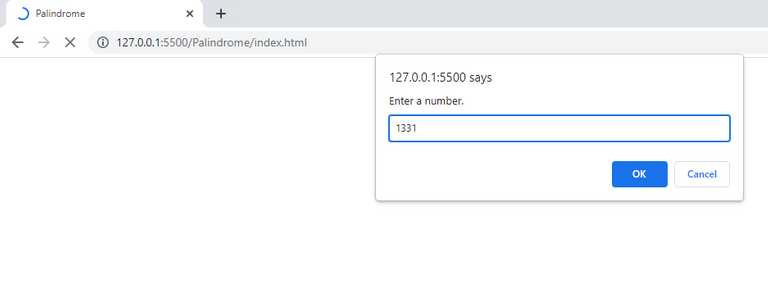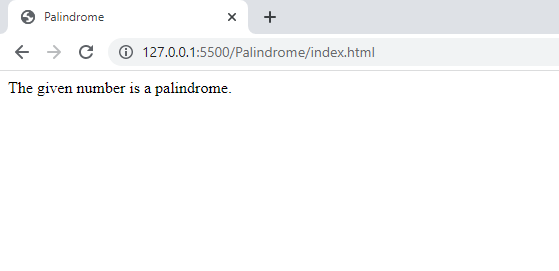# Checking For A Palindrome Number In JavaScript

in STEMGeeks2 years agoImage Source

Before starting to write a program to check for a palindrome number, first lets know what palindrome numbers are. They are those number that are same while reading from left to right and right to left. The same concept goes for palindrome string as well. For example: "MADAM" is a palindrome word and "12121" is a palindrome number. If you just know the simple logic to check for the palindrome number then it is easy to write the code with while-loop and if-else condition syntax. The simple logic to achieve this is that we will find the quotient and remainder of the given number when divided by ten and at each iteration again we will find the remainder and quotient when previous quotient is divided by ten. We will continue this until the quotient value is 0. The same logic is applied in the code below:

``````<!DOCTYPE html>
<html>
<title>Palindrome</title>
<script>
var x = prompt("Enter a number.");
var temp = x;
var y = "";
var q = parseInt(x/10);
while (temp!= 0)
{
var r = temp % 10;
y = y + r;
temp=q;
q = parseInt(temp/10);
}
if (x==y)
{
document.write("The given number is a palindrome.")
}

else{
document.write("The given number is not a palindrome.")
}
</script>
<body>
</body>
</html>
``````

If you run the above script in your browser you will first get a pop up dialog box with a text area field to input a number.After you entered your desired number and press ok, then it will show the following output. You can see by using `prompt()` function, the browser keeps on reloading unless you provide the value. You can use it now for learning purposes. But in real life project it is a bad design as your browser UI remains hidden unless you enter the value in the text-field.